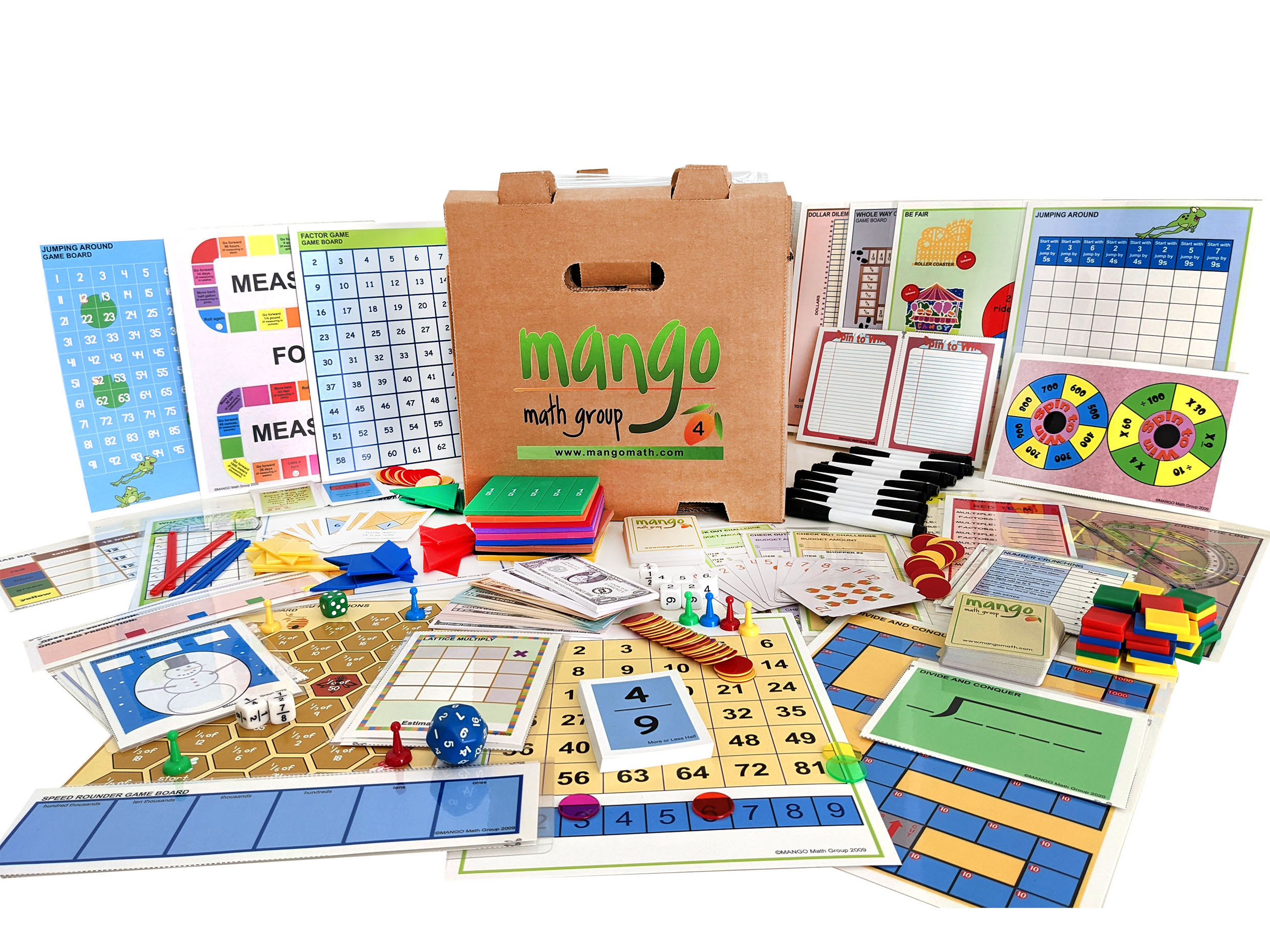#### Order Notice

As the economy opens back up, many of our suppliers are experiencing shortages of parts and raw materials, resulting in delays in shipments. As a result, we anticipate that it may take up to 2-4 weeks from the date we receive a purchase order for us to fulfill and ship any order for our products. We apologize for any inconvenience.

# Fourth Grade Deluxe Math Kit

Exploring fourth grade math skills has never been easier and more fun! MANGO Math's Strong Evidence Based Deluxe Fourth Grade Math Kit contains 20 separate skill building math lessons.

\$ 459.00 USD##### Product Details

MANGO Math's Fourth Grade Deluxe Math Kit includes 20 individually packaged games that help fourth graders develop understanding and fluency of multi-digit multiplication and division of whole numbers with the understanding of place value and properties of operations.

Each individual lesson offers visual and kinetic representation of math concepts that develop strong math knowledge, like:

• fraction equivalence
• additional and subtraction of fractions with like denominators

Every lesson is based on a game or activity that fosters curiosity, discussion and growth through cooperation, collaboration and creative thinking.

MANGO Math's Strong Evidence Based Kits come with individually packaged, Grab-n-Go lessons that includes instructions, reusable materials pre-packaged in a resealable pouch contained in an easy to carry crate that can be used year after year, providing long term savings.

A lesson can be used by up to 4 students, set up as math centers with each grade level math kit meeting the needs of 30+ students at a time.

Each MANGO Math grade level math kit is research-based and adheres to Common Core, TEKS and NCTM math standards.

#### All the supplies below are included with its specific lesson in the kit:

• Spinners
• 6-sided dice
• 10-sided dice
• Fraction dice
• Tens dice
• Hundreds dice
• Thousands dice
• Place value dice
• Transparent counters
• Colored tiles
• Play money: dollars and coins
• Anglegs
• Protractors
• Measuring tape
• MANGO playing cards
• Bi-colored counters
• Fraction tiles
• Stacking counters
• Game pawns
• Paper bags
• Resealable plastic pouch
• Game boards
• Laminated writing boards
• Dry erase markers
• Curriculum Guide

## Fourth Grade Lessons with Standards

Check Out Challenge – Measurement: Use the addition and subtraction to solve word problems involving money including problems involving simple decimals.

Measure for Measure – Measurement: Know relative sizes of measurement units within one system of units. Express measurements in a larger unit in terms of smaller unit.

Tricky Tangrams – Measurement: Apply the area formulas in real-world mathematical problems, using simple fractions.

Be Fair – Algebraic Thinking & Operations: Multiply or divide to solve word problems involving multiplicative comparison, use symbol for the unknown    number.

Dollar Dilemma – Algebraic Thinking & Operations: Solve multistep word problems posed with whole numbers and having whole-number answers using the four operations.

Factor Game – Algebraic Thinking & Operations: Find all factor pairs for a whole number in the range 1—100.  Recognize multiple of each of its factors.

Jumping Around – Algebraic Thinking & Operations: Generate a number that follows a given rule.  Identify apparent features of the pattern that were not explicit in the rule itself.

Tic-Fact-Toe – Algebraic Thinking & Operations: Find all the factor pairs for a whole number in the range 1—100.  Recognize that a whole number is a multiple of each of its factors.

Combing Thru Fractions – Number Operations & Fractions: Solve problems involving multiplication of a fraction by a whole number using visual fraction models.

Divide & Conquer – Number Operation & Base Ten: Find Whole-number quotients and remainders with up to 4-digit dividends & 1-digit divisors

Frozen Fraction Friends – Number Operations & Fractions: Understand addition and subtraction of fractions as joining and separating parts referring to the same whole.

Lattice Multiply – Number Operations & Base Ten: Multiply a whole number of up to 4-digits by a 1-digit whole number, using strategies based on place value.

More or Less Half - Number Operations & Fractions: Compare two fractions with different numerators and different denominators by comparing to a benchmark fraction like 1/2.

Number Crunching – Number Operations & Base Ten: Compare two decimals to hundredths by reasoning about their size.

Speed Rounder – Number Operations & Base Ten: Use place value understanding to round multi-digit whole numbers to any place.

Spin to Win – Number Operations & Base Ten: Recognize that in a multi-digit whole number, a digit in one place represents 10 times what is represents in the place to the right.

Whole Way Out – Number Operations & Fractions: Understand addition and subtraction of fractions as joining and separating parts referring to the same whole.

Cross the Line – Geometry: Classify two-dimensional figures based on the presence or absence of angles, perpendicular and parallel lines.

What’s Your Angle – Geometry: Understand concepts of angle and measurement angle.

Grab Bag – Odds & Order: Solve word problems involving addition and subtraction of fractions referring  to the same whole and having like denominators.

Width
13
in
Height
13
in
Length
19
in
Weight
20
lb